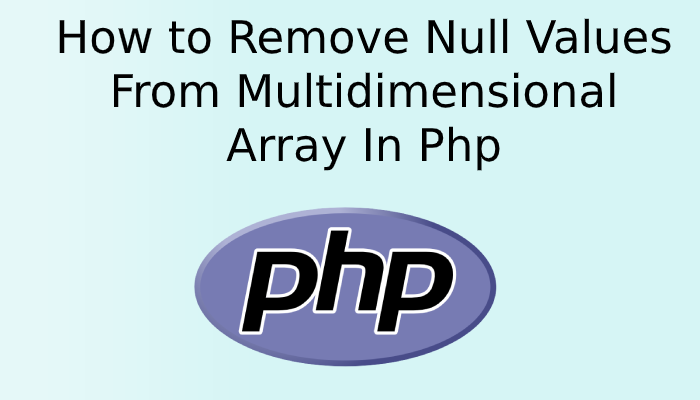# How to Remove Null Values From Multidimensional Array In PhpLet’s see how we can remove null values from a multidimensional array in PHP. We have provided two examples and tried to make you understand, How to Remove Null Values From Multidimensional Array In Php.

We have two methods, by using them we can remove the NULL value from the array in PHP.

array_filter() is an array method in PHP which remove the null values from array.

In the second method, we can check run a foreach loop and check if any null value present in the array and remove it using unset().

So, let’s see the below example of remove null in multidimensional array in PHP.

Example 1:

```<?php

\$students = [
0 => [
'id' => 1,
'email' => '[email protected]'
],
1 => [
'id' => 2,
'email' => '[email protected]'
],
2 =>null,
3 => [
'id' => 4,
'email' => '[email protected]'
],
4 => null,
];
\$students = array_filter(\$students);
print_r(\$students);

?>```

Output :

``````Array
(
 => Array
(
[id] => 1
[email] => [email protected]
)

 => Array
(
[id] => 2
[email] => [email protected]
)

 => Array
(
[id] => 4
[email] => [email protected]
)

)``````

Example 2:

```<?php

\$user = [

0 => [
'id' => 1,
'email' => '[email protected]'
],
1 => [
'id' => 2,
'email' => '[email protected]'
],
2 => null,
3 => [
'id' => 5,
'email' => '[email protected]'
],

];

foreach (\$user as \$key=>\$val) {
if (\$val === null)
unset(\$user[\$key]);
}
print_r(\$user);
?>```

Output :

``````Array
(
 => Array
(
[id] => 1
[email] => [email protected]
)

 => Array
(
[id] => 2
[email] => [email protected]
)

 => Array
(
[id] => 5
[email] => [email protected]
)

)``````